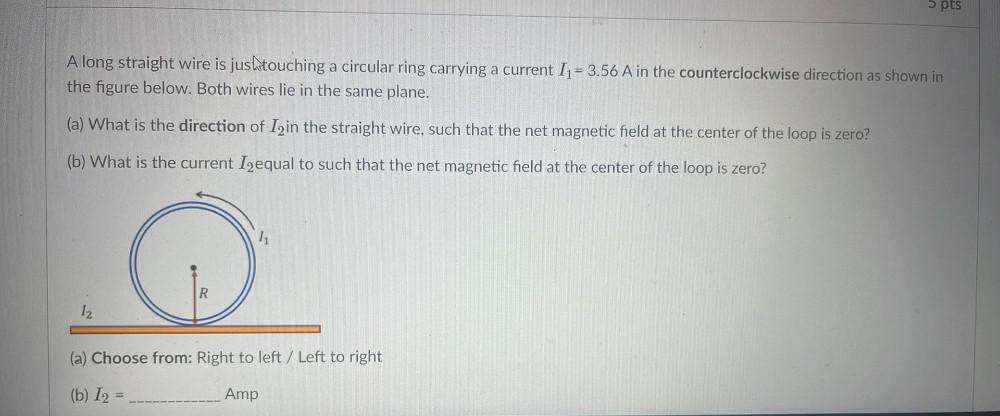Question:

# 5 pts A long straight wire is jushtouching a circular ring carrying a current I1 = 3.56 A in the counterclockwise direction as s5 pts A long straight wire is jushtouching a circular ring carrying a current I1 = 3.56 A in the counterclockwise direction as shown in the figure below. Both wires lie in the same plane. (a) What is the direction of Izin the straight wire, such that the net magnetic field at the center of the loop is zero? (b) What is the current I2equal to such that the net magnetic field at the center of the loop is zero? R 12 (a) Choose from: Right to left / Left to right (b) 12 = Amp